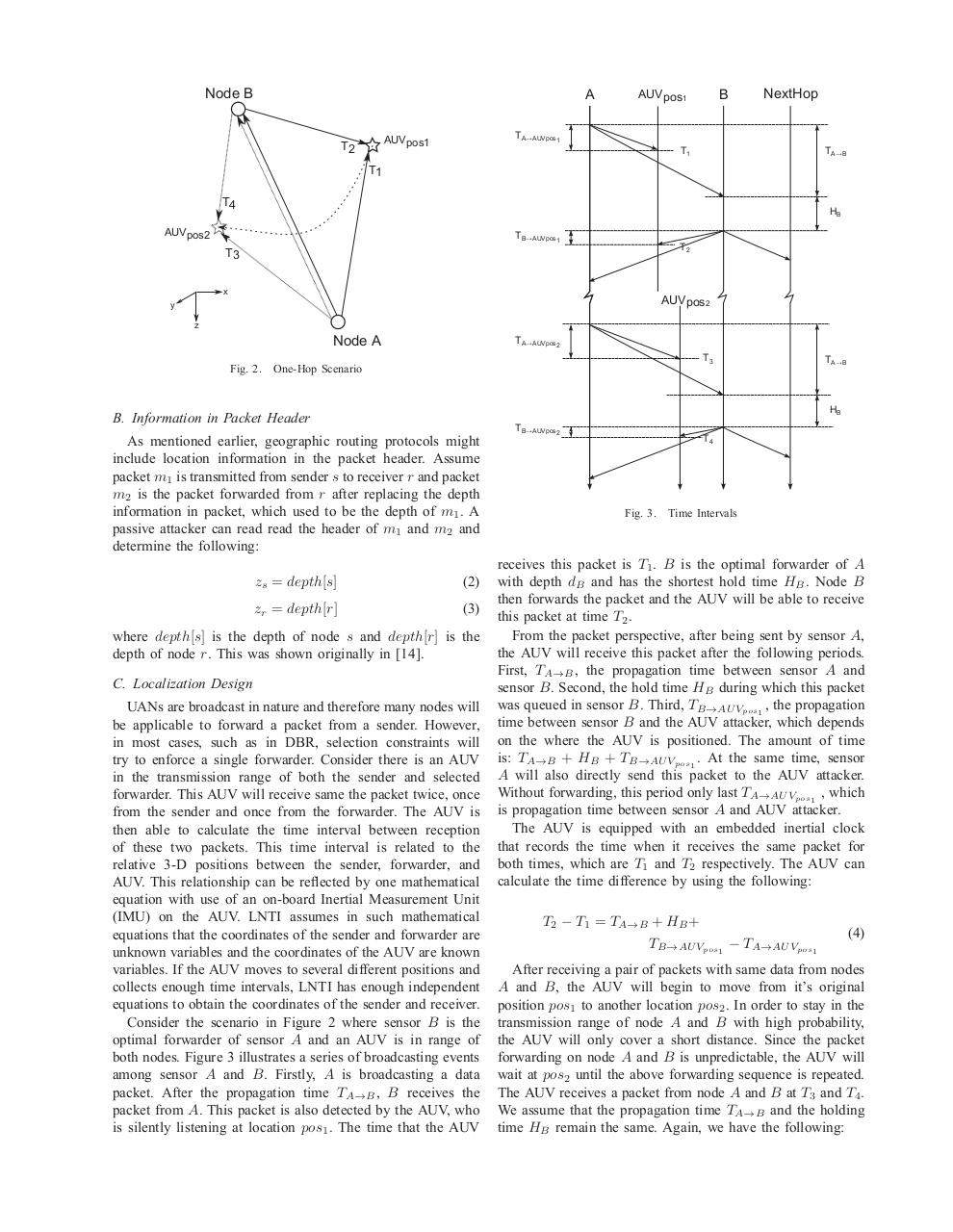# 1569926793.pdfPage 1 2 3 4 5 6 7 8 9

#### Text preview

Node B

A

NextHop

TA✁AUVpos1

AUVpos1

T2

B

AUVpos1

TA✁B

T1

T1
T4

HB

AUVpos2

TB✁AUVpos1

T2

T3
x

AUVpos2

y
z

TA✁AUVpos2

Node A
Fig. 2.

T3

One-Hop Scenario

TA✁B

HB

As mentioned earlier, geographic routing protocols might
include location information in the packet header. Assume
packet m1 is transmitted from sender s to receiver r and packet
m2 is the packet forwarded from r after replacing the depth
information in packet, which used to be the depth of m1 . A
determine the following:
zs = depth[s]

(2)

zr = depth[r]

(3)

where depth[s] is the depth of node s and depth[r] is the
depth of node r. This was shown originally in .
C. Localization Design
UANs are broadcast in nature and therefore many nodes will
be applicable to forward a packet from a sender. However,
in most cases, such as in DBR, selection constraints will
try to enforce a single forwarder. Consider there is an AUV
in the transmission range of both the sender and selected
forwarder. This AUV will receive same the packet twice, once
from the sender and once from the forwarder. The AUV is
then able to calculate the time interval between reception
of these two packets. This time interval is related to the
relative 3-D positions between the sender, forwarder, and
AUV. This relationship can be reﬂected by one mathematical
equation with use of an on-board Inertial Measurement Unit
(IMU) on the AUV. LNTI assumes in such mathematical
equations that the coordinates of the sender and forwarder are
unknown variables and the coordinates of the AUV are known
variables. If the AUV moves to several different positions and
collects enough time intervals, LNTI has enough independent
equations to obtain the coordinates of the sender and receiver.
Consider the scenario in Figure 2 where sensor B is the
optimal forwarder of sensor A and an AUV is in range of
both nodes. Figure 3 illustrates a series of broadcasting events
among sensor A and B. Firstly, A is broadcasting a data
packet. After the propagation time TA→B , B receives the
packet from A. This packet is also detected by the AUV, who
is silently listening at location pos1 . The time that the AUV

TB✁AUVpos2

T4

Fig. 3.

Time Intervals

receives this packet is T1 . B is the optimal forwarder of A
with depth dB and has the shortest hold time HB . Node B
then forwards the packet and the AUV will be able to receive
this packet at time T2 .
From the packet perspective, after being sent by sensor A,
the AUV will receive this packet after the following periods.
First, TA→B , the propagation time between sensor A and
sensor B. Second, the hold time HB during which this packet
was queued in sensor B. Third, TB→AU Vpos1 , the propagation
time between sensor B and the AUV attacker, which depends
on the where the AUV is positioned. The amount of time
is: TA→B + HB + TB→AU Vpos1 . At the same time, sensor
A will also directly send this packet to the AUV attacker.
Without forwarding, this period only last TA→AU Vpos1 , which
is propagation time between sensor A and AUV attacker.
The AUV is equipped with an embedded inertial clock
that records the time when it receives the same packet for
both times, which are T1 and T2 respectively. The AUV can
calculate the time difference by using the following:
T2 − T1 = TA→B + HB +

TB→AU Vpos1 − TA→AU Vpos1

(4)

After receiving a pair of packets with same data from nodes
A and B, the AUV will begin to move from it’s original
position pos1 to another location pos2 . In order to stay in the
transmission range of node A and B with high probability,
the AUV will only cover a short distance. Since the packet
forwarding on node A and B is unpredictable, the AUV will
wait at pos2 until the above forwarding sequence is repeated.
The AUV receives a packet from node A and B at T3 and T4 .
We assume that the propagation time TA→B and the holding
time HB remain the same. Again, we have the following: